# Magnetic Flux vs Temperature Relationship in Ferromagnets

Hi everyone,

I have recently done an experiment testing the effect of temperature on magnetism through measurement of magnetic flux at a constant distance away from the measuring device. I used a range of 0-90 C for neodymium and bar magnets, and found a reasonably linear trend, with a similar slope to what I was expecting from its coefficient of residual induction.

However, when I inserted linear trendlines for my data, they gave an unreasonably high curie point (when there was 0 flux density) for the bar magnets, indicating the trend could not have been linear in order for the flux density to be 0 at the Curie point. Even more perplexing was the fact that the relationship DID seem to be linear for the neodymium magnets!

After I did some more research, I found that the temperature coefficient of residual induction was not necessarily linear, especially outside of the 0-100 C range. Does anyone have formulas which describes this change, or formulas for the relationship between magnetic flux density and temperature? I've researched for hours, but the best relationship I could come up with was the linear approximation from the temperature coefficient of residual induction.

•astrocat98

Homework Helper
Gold Member
In addition, I believe general formulas like this in regards to phase transitions are covered in the book by Nigel Goldenfeld on the Renormalization Group: https://www.amazon.com/dp/0201554097/?tag=pfamazon01-20 The renormalization group theory actually predicts such an equation with the exponent ## \gamma=.32 ##, etc., with the 3-D Ising model. (see p.111 of the book=I have a copy of this book and it contains some very interesting calculations and info.)
@vanhees71 and @blue_leaf77 . A very interesting topic that the OP posted. Might you have any additional inputs?

Last edited:
By additional inputs, do you mean did I measure any other variables other than magnetic flux? Unfortunately, I didn't, and wish I had in hindsight. How did you find the equation that you posted above [M/Mo = ((Tc-T)/Tc)^0.36]? The picture of the graph doesn't even give a formula. Could you please send me the information on the renormalization group theory and the equation? I don't actually have the Nigel Goldenfeld book.

Would I be able to calculate a value of y from my datasets, or should I just use the approximation of y = 0.32 given by the book? I can see how you can convert B to H by using M = Mr x Mo and substituting M into B = M x H, but how would I find values of B outside my measured temperature range (0-90 C)? Is this covered in the book as well?

Homework Helper
Gold Member

Last edited:
Thanks so much! This has really helped. One last thing - is M0 the magnetisation at T = 0, or is it something else entirely? Would I be able to find out what M0 is for my dataset?

I have M = µ0 x Br
so Br = M/µ0

and B =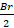(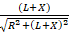-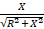) for my cylindrical neodymium magnet, where L = length of magnet, R = radius of magnet and X = distance from pole, all in mm.
so B =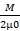(-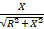)

From the relationship you gave me, M/M0 = ((Tc-T)/Tc)^0.36.

I know what temperatures I measured the flux density at, so I can find what fraction of the Curie temperature that is and use the graph to find what M/M0 was for that temperature. How do I find M0 so I can substitute it into M/M0 = ((Tc-T)/Tc)^0.36, to find M, then substitute M into my flux formula?. Could I find what M0 is using T/Tc = 0?

#### Attachments

•Homework Helper
Gold Member
Very good. Yes, ## M_o ## is the magnetization of the permanent magnet at ## T=0 ## K, and I'm pretty sure, at least for best results, the geometry needs to be a long cylindrical shape. (and not spherical, etc.). Your data point at the lowest temperature you measured will be reasonably close to this value. ## \\ ## ( ## M_o ## for the material should also be available in a google. Let me see if it shows up... They give 1.6 T, but that is for a very high quality special compound ## Nd_2Fe_{14}B ##. If you got something in the neighborhood of 1.0 T, it is probably reasonably accurate...Note: For units, use ## B=\mu_o H+M ##, so that ## B_r=M ##. That's the simplest wat to compute it. (Sometimes the equation ## B=\mu_o H +\mu_o M ## is also used=this makes ## M=B_r/\mu_o ##. Your ## M=\mu_o B_r ## is incorrect) . ## \\ ## Yes, you could also extrapolate back to ## T/T_C=0 ##. The one problem you will likely have is you didn't go to extremely high temperatures. Note: My computer was unable to read the .png data sets. I'm very curious to know though, what did you get for ## B_r=M ##? ## \\ ## One additional item you might find of interest, since you are on the subject of magnetism is a PhysicsForums Insights article that I recently authored. https://www.physicsforums.com/insights/permanent-magnets-ferromagnetism-magnetic-surface-currents/ I didn't go into a discussion of the quantum mechanical exchange effect in this article, but it might give you some introduction into permanent magnets.

Last edited:
I have been working through this Experiment with @Scoopadifuego and we are both very, very greatful for your help

•Does anyone know of any values of magnetism (mT) at 0K for neodymium and Iron magnets?

Homework Helper
Gold Member
Does anyone know of any values of magnetism (mT) at 0K for neodymium and Iron magnets?
Try this "link": https://en.wikipedia.org/wiki/Remanence ## \\ ## I'm curious=what did you get when you computed ## B_r=M_o ## from your data?

By substituting Br = Mμ0 and M = M0 ((Tc - T)/Tc)^0.36 into B =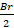(-) and rearranging, I got M0 = 0.1233T, but it seems too low, as you mentioned above it should be somewhere around 1T. This would give Br = 1.55 x 10^-7 ((583.15-T)/583.15)^0.36, which doesn't make sense at all, as Br should be about 1T for neodymium magnets. Also, when I substitute the value of M0 into my flux formula, it gives B = 1.12 x 10^-10 (((583.15-T)/583.15)^0.36, which is thousands of times lower than what my data actually is.

I've checked the calculations multiple times and can't see anything wrong, so I'm figuring I've messed up somewhere in constructing my formula.

Homework Helper
Gold Member
By substituting Br = Mμ0 and M = M0 ((Tc - T)/Tc)^0.36 into B = View attachment 209841 ( View attachment 209842 - View attachment 209843 ) and rearranging, I got M0 = 0.1233T, but it seems too low, as you mentioned above it should be somewhere around 1T. This would give Br = 1.55 x 10^-7 ((583.15-T)/583.15)^0.36, which doesn't make sense at all, as Br should be about 1T for neodymium magnets. Also, when I substitute the value of M0 into my flux formula, it gives B = 1.12 x 10^-10 (((583.15-T)/583.15)^0.36, which is thousands of times lower than what my data actually is.

I've checked the calculations multiple times and can't see anything wrong, so I'm figuring I've messed up somewhere in constructing my formula.
What did you measure for ## B ## at a distance from one of the pole faces of the magnet? Also, what is the ## L ## for the magnet, and how far was your distance ## X ## from the pole endface?

•Lussimio16
B = 3.47 x 10^-4 T at 20 degrees C, so 293.15K at about 25mm away from the meter, where L = 39mm and R = 3mm.

Homework Helper
Gold Member
The ## M_o=B_r=.12 ##T looks sort of ok. I think you got the arithmetic correct=I estimated it, but didn't compute it with a calculator. I presume in the data you gave me that ## X=25 ## mm. It might not be a very high quality magnet that you have. Meanwhile, the formula reads ## B_r=M_o ## without any ## \mu_o ##, at least in the units I have been using. If you write ## .12=M_o (1-295/583)^{.36}=M_o (.8) ##(approximately), so that ## M_o=.15 ## T which could be reasonable for a low quality Neodynium magnet. It could perhaps also be due to errors in the measurement of the magnetic field ## B ##.

@Scoopadifuego, the Neodymium magnet we used was pretty low quality in comparison to other Neodymium magnets so this is actually quite justifiable.

•Homework Helper
Gold Member
From all appearances, your experiment was pretty successful. I don't know how accurate your meter is that measured the magnetic field strength, but if it was accurate, then you might simply have a Neodynium magnet of only moderate strength. Your data collection and formulas look good. :)

I'd just like to extend my appreciation for your help again. You have been fantastic ! Thank you for your guidance :) @CharlesLink

•Homework Helper
Gold Member

Last edited:
@Charles Link Thank you so much for helping @zac_physics_student and me with this problem. The graphs turned out well, and I wouldn't have been able to do this without your help.

#### Attachments

•Homework Helper
Gold Member
Homework Helper
Gold Member
@zac_physics_student and @Scoopadifuego I decided to give the compass measurement idea a try that I mentioned in post #18. I went out and bought a compass and some (a stack of 15) strong disc magnets (they were inexpensive) from which I made a cylindrical bar magnet that was 2.75" long and ## D= 11/16 ##" in diameter. With the magnet at a distance of 14" from the compass, the compass needle was pulled westward by 45 degrees, and likewise, upon turning the magnet around, the compass needle was pushed eastward by 45 degrees at the same distance. ## \\ ## The pole method made for simple calculations: (Alternatively, you could use the formula you did that is derived from the magnetic surface currents. A Taylor series expansion of the denominators with the square roots in your expression with ## L ## and/or ## X>>R ## would give the very same formula for ## H ## and ## B ## that I have here.) ## \\ ## ## \sigma_m=M ## and ## A=\pi D^2/4 ##. ## \\ ## Meanwhile ## H=\frac{1}{4 \pi \mu_o} \sigma_m A(\frac{1}{s_1^2}-\frac{1}{s_2^2} ) ## where ## s_1=14##" and ##s_2=16.75 ##". ## \\ ## Using ## B=\mu_o H =.5 E-4 ## T,(.5 gauss=.5 E-4 T is the approximate strength of the Earth's magnetic field), gives the result that ## M=1.1 ## T.## \\ ## *********************************************************************************************************************************************************** ## \\ ## And one additional note: The Taylor series calculation is ## \frac{L+X}{\sqrt{(L+X)^2+R^2}}=\frac{1}{\sqrt{1+(\frac{R}{L+X})^2}} \approx 1-\frac{1}{2}(\frac{R}{L+X})^2 ## and similarly for the term without any ## L ##. The result is that this calculation for the on-axis ## B ## from the magnetic surface currents agrees with the on-axis ## B ## computed by the pole method in the approximation that the poles can be treated like point sources. ## \\ ## ******************************************************************************************************************** ## \\ ## Editing: Additional input: The value that I used for the Earth's magnetic field as .5 gauss in my location might be somewhat high. The official value they have on-line for the north horizontal component is only .2 gauss and the vertical component is .5 gauss, but only the horizontal component will affect the compass when placed horizontal. Thereby, the ## M ## of the magnet that I used, found by computation, would be approximately .4 T rather than 1.1 T.

Last edited:
•zac_physics_student and vanhees71
Lussimio16
The ## M_o=B_r=.12 ##T looks sort of ok. I think you got the arithmetic correct=I estimated it, but didn't compute it with a calculator. I presume in the data you gave me that ## X=25 ## mm. It might not be a very high quality magnet that you have. Meanwhile, the formula reads ## B_r=M_o ## without any ## \mu_o ##, at least in the units I have been using. If you write ## .12=M_o (1-295/583)^{.36}=M_o (.8) ##(approximately), so that ## M_o=.15 ## T which could be reasonable for a low quality Neodynium magnet. It could perhaps also be due to errors in the measurement of the magnetic field ## B ##.
What is Br?
I am doing a similar experiment for an IB assessment, but it turned out to be more complicated than I thought... I measured the field strength of a magnet cooling down from 80C to room temp, but I am struggling with how to process the data for the lab report

Last edited:
Homework Helper
Gold Member
What is Br?
I am doing a similar experiment for an IB assessment, but it turned out to be more complicated than I thought... I measured the field strength of a magnet cooling down from 80C to room temp, but I am struggling with how to process the data for the lab report
This should give you a good introduction into the various parameters involved in the magnetic field from a magnetized cylinder.

Edit: What does your data look like? Some detail might help in determining what you need to do to process it. With Curie temperatures ## T_C ## that can be above 1000 degrees C, a temperature of 80 degrees C may or may not give much change in the magnetic field from room temperature. See especially posts 2 and 3 above.

Last edited:
•vanhees71
Person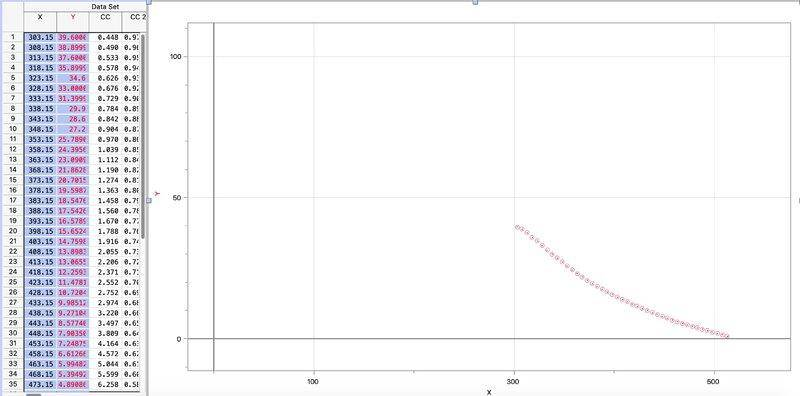This is data I took from an NdFeB magnet where temperature in K is on the x and B in millitesla is on the y. The data doesn’t seem to fit what you all are saying and I wanted to get y’all’s opinions.

•Person
In this experiment the magnet is kept at a fixed distance away from the gaussmeter.

•Homework Helper
Gold Member
The data looks good. Looks like the Curie temperature ## T_C ## is around 500K. The theoretical formula in post 2, ## M=M_o ((T_C-T)/T_C))^{\gamma} ## , would have a more rapid decline at the Curie temperature (see the graphs of post 19), but the theoretical formula may not be exact.

Just an additional comment: The mechanism for a permanent magnet is a rather complex one, and it shouldn't be too surprising if there are some differences between experiment and the formula that comes from group theory and critical phenomena.

One more item: a google gives ## T_C=583K ## for NdFeB magnets, so your data is certainly in the right ballpark.

Last edited:
•vanhees71 and Person
Homework Helper
Gold Member
In response to a question from @IBphysics There are basically 2 different types of MKS units for the magnetization ## M ## in common use: The older textbooks like to use the formula ## B=\mu_o H+M ##, while the newer textbooks seem to be switching to ## B=\mu_o H+\mu_o M ##. For the first formula, ## M ## has units of ## T ##, while the second one will have units of ## A/m ##.

I prefer using ## T ##, but some others prefer the ## A/m ##.

Last edited:
•vanhees71